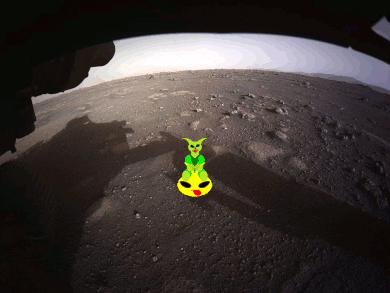-=+=- -=+=- -=+=- -=+=- -=+=- -=+=- -=+=- -=+=- -=+=- -=+=- -=+=- -=+=- -=+=- -=+=- -=+=- -=+=- -=+=- -=+=- -=+=- -=+=- -=+=- -=+=- -=+=- -=+=- -=+=- -=+=- -=+=- -=+=- -=+=- -=+=- (c) WidthPadding Industries 1987 0|132|0 -=+=- -=+=- -=+=- -=+=- -=+=- -=+=- -=+=- -=+=- -=+=- -=+=- -=+=- -=+=- -=+=- -=+=- -=+=- -=+=- -=+=- -=+=- -=+=- -=+=- -=+=- -=+=- -=+=- -=+=- -=+=- -=+=- -=+=- -=+=- -=+=- -=+=-
Socoder -> Off Topic -> Alien spotted on Mars

Posted : Saturday, 20 February 2021, 00:37
steve_ancell### Alien spotted on Mars

It had to be done, I just couldn't help myself. Bordom must be rotting my brain to the point where I need to make bouncy alien on Mars GIFs and may be in need of a white jacket that has wrap-around sleeves and buckles-up at the back.